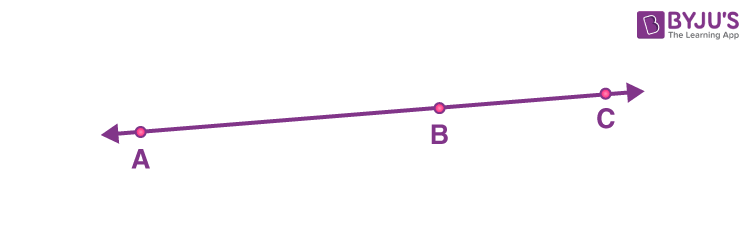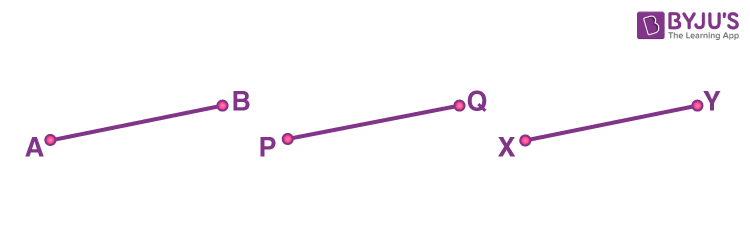# Introduction to Euclid’s Geometry Class 9 Notes

Introduction to Euclid’s Geometry class 9 notes is given here for students to attain good marks in the examination. We know that Geometry is one of the branches of Mathematics and the term “Geometry” is derived from the Greek words “Geo”, which means earth and “Metron” which means measurements. Geometry deals with the study of properties of different shapes. In this article, we are going to discuss Euclid’s approach to Geometry and his definitions, axioms, and postulates in detail.

## Class 9 Maths Chapter 5 Introduction to Euclid’s Geometry Notes

We know that a solid has a size, shape and position, which can be moved from one position to another position. The boundaries of solids are called surfaces and they have no thickness. Similarly, the boundaries of surfaces are curves or straight lines. These lines should end in points. Hence, the steps from solid to points are solids – surfaces- lines-points. Here, in each step, we lose one extension called dimension. It means:

• Solids – 3 dimension
• Surface – 2 dimension
• Line – 1 Dimension
• Point – No dimension or zero dimension

From these observations, Euclid summarised these statements as definitions. Now, let us discuss some of Euclid’s definitions.

### Euclid’s Definition

Some definitions of Euclid’s given in Book 1 of the “Elements” are:

1. The ends of a line are points.
2. A line is a breadthless length.
3. A point is that which has no part.
4. The edges of a surface are lines.
5. A surface is that which has length and breadth only.
6. A plane surface is a surface which lies evenly with the straight lines on itself.
7. A straight line is a line which lies evenly with the points on itself.

### Euclid’s Axioms

Using his definition, Euclid assumed some properties, which were not to be proved. Those assumptions are obvious universal truths. Euclid divided them into two parts called axioms and postulates. Some of Euclid’s axioms are:

1. Things which are equal to the same thing are equal to one another.
2. If equals are added to equals, the wholes are equal.
3. If equals are subtracted from equals, the remainders are equal.
4. Things which coincide with one another are equal to one another.
5. The whole is greater than the part.
6. Things which are double of the same things are equal to one another.
7. Things which are halves of the same things are equal to one another.

### Euclid’s Five Postulates

The five postulates of Euclid’s are:

Euclid’s Postulate 1: A straight line may be drawn from any one point to any other point.

Euclid’s Postulate 2: A terminated line can be produced indefinitely.

Euclid’s Postulate 3: A circle can be drawn with any centre and any radius.

Euclid’s Postulate 4: All right angles are equal to one another.

Euclid’s Postulate 5: If a straight line falling on two straight lines makes the interior angles on the same side of it taken together less than two right angles, then the two straight lines, if produced indefinitely, meet on that side on which the sum of angles is less than two right angles.

After Euclid stated his axioms and postulates, he used them to prove the result of some statements. The mathematical statement whose truth has been proved is called a theorem or proposition.

Now, let us discuss how to prove a theorem using axioms.

Theorem: Two distinct lines cannot have more than one point in common.

Proof: Consider two lines, say l and m. Now, we have to prove that they have only one point in common.

Suppose two lines intersect at two distinct points, say A and B. Hence, we have two lines passing through two distinct points A and B. But this assumption clashes with Euclid’s axiom that “only one line can pass through two distinct points”.

Hence, we can conclude that two distinct lines cannot have more than one point in common.

### Equivalent Version of Euclid’s Fifth Postulate

Euclid’s fifth postulate plays a very significant role in Mathematics. There are many equivalent versions of Euclid’s fifth postulate. Two of them are:

1. For every line l and for every point P not lying on l, there exists a unique line m passing through P and parallel to l.
2. Two distinct intersecting lines cannot be parallel to the same line.

## Introduction To Euclid’s Geometry Class 9 Examples

Example 1:

Let A, B and C be the three points on the line. Point B lies between A and C. Prove that AB + BC = AC.Solution:

In the given figure, line segment AC coincides with AB+BC.

By using Euclid’s axiom, “Things which coincide with one another are equal to one another”, we can say AB+BC = AC.

Hence, the statement “AB+BC = AC” is proved.

Example 2:

In the given figure, if AB = PQ, and PQ = XY, then AB = XY.Solution:

The statement, “ if AB = PQ, and PQ = XY, then AB = XY” is “True”.

According to the Euclid axiom, “the things which are equal to the same thing are equal to one another”, the given statement is true.

### Practice Problems

Solve the following problems of class 9 Maths chapter 5 introduction to Euclid’s geometry.

1. If two circles are equal, then their radii are equal. Is this statement true or false? Also, justify your answer.
2. There is an infinite number of lines that pass through two distinct points. Is this statement true or false? Also, justify your answer.
3. Assume that point C lies between two points, say A and B, such that AC = BC. Prove that AC = ½ AB.

Stay tuned with BYJU’S – The Learning App and download the app today to get all class-wise concepts.